• MatLab实现SVM分类
千次阅读
2021-04-23 09:45:17

在当前数据挖掘和机器学习领域，最为热门的话题莫过于SVM和Boosting方法了。只要是涉及到这两个主题，那么论文就会容易被杂志和会议接受了。看来不管做什么，都讲究眼球效应啊。搞研究其实也有点类似超级女声，呵呵。

以前我的论文中用的SVM Code都来自于台湾的林智仁教授的LibSVM。真的是佩服有些大家，自己做出了重要的发现和成果，想到的不是把自己的成果保密起来(像C4.5一样)，让自己独享自己的成果；而是让自己的成果最大程度的被人所利用，给后来的研究人员打下一个坚实的基础。说实话，他的代码很好，用起来很方便，而且不同的语言版本实现都有，即使是对于初学者都很容易掌握。不过用的久了，我就想自己也实现SVM，现在的数学计算工具太多了，而且功能齐全，用起来方便。今天鼓捣了一会，终于用Matlab实现了第一个SVM。虽然比较简单，但是包含了大多数SVM的必要步骤。

这个实现是线性可分支持向量分类机，不考虑非线性分类引入核函数的情况，也不考虑推广条件下引入Penalty Loss的情况。

问题描述：平面上有如下点A = [1 1.5;2 1.5;3 1.5;4 1.5;1 0.5;2 0.5;3 0.5;4 0.5]及其对应的标号flag = [1 1 1 1 -1 -1 -1 -1];用SVM方法构造一个决策函数实现正确分类。

如果我们在二维坐标上描点，就会发现这是个很简单的线性可分问题。实现方法，用SVM 的对偶问题，转换为Matlab的有约束非线性规划问题。

构建m文件：

function f = ffsvm(x)

A = [1 1.5;2 1.5;3 1.5;4 1.5;1 0.5;2 0.5;3 0.5;4 0.5];

flag = [1 1 1 1 -1 -1 -1 -1];

for i=1:1:length(A)

for j=1:1:length(A)

normA(i,j) = A(i,:)*A(j,:)';

normFlag(i,j) = flag(1,i)*flag(1,j);

end

end

f = 0;

for i=1:1:length(A)

for j=1:1:length(A)

f = f + 1/2*(normA(i,j)*x(i)*x(j)*normFlag(i,j));

end

f = f - x(i);

end

在命令窗口输入：

Aeq = [1 1 1 1 -1 -1 -1 -1];

beq = 0;

lb = [ 0 0 0 0 0 0 0 0];

调用MatLab内置优化函数fmincon；

更多相关内容
• 该代码包括了SVM的多核核函数的分类实现和可视化展示。
• 该代码包括了SVM的多核核函数的分类实现和可视化展示。
• 基于MATLABsvm分类器代码实现基于MATLABsvm分类器代码实现
• 提供的 MATLAB 函数可用于使用基于树状图的支持向量机 (D-SVM) 对数据集进行训练和执行多类分类。 两个主要功能是： Train_DSVM：这是用于训练的函数Classify_DSVM：这是用于 D-SVM 分类的函数示例：使用fisheriris ...
• 它有效解决了接触式疲劳检测方法给驾驶员带来的干扰以及单一信号源对于反映疲劳程度可靠性低的问题，同时通过设计神经网络模型对多源信息进行分类实现对疲劳状态的高精度和高速度的检测。选取合适的特征值对网络...
• 内含libsvm工具箱、SVMs的示例程序（含代码和实例数据）、SVR的示例程序（含代码和实例数据）
• SVM神经网络中的参数优化---提升分类器性能(matlab实现)，包含源代码和测试数据
• 利用MATLAB实现SVM分类 %% LibSVM clc;clear;close all; %% 加载文件 [Iris1,Iris2,Iris3,Iris4,IrisClass]=textread('iris.data','%f%f%f%f%s','delimiter',','); Wine=load('wine.data'); % 仅读数字 Abalone=...

## 利用MATLAB实现SVM分类

%% LibSVM
clc;clear;close all;
%% 加载文件
Abalone=importdata('abalone.data');%既可以读取数据又可以读取字符
%% 数据预处理
% Iris数据预处理
Iris_all=[Iris1,Iris2,Iris3,Iris4];
Iris_class = zeros(1, 150);
for i = 1: size(IrisClass,1)
if (strcmp(IrisClass(i), 'Iris-setosa' ))
Iris_class(1,i) = 1;
elseif(strcmp(IrisClass(i), 'Iris-versicolor') )
Iris_class(1,i) = 2;
else
Iris_class(1,i) = 3;
end
end
Iris_class=Iris_class(:);
%------数据类别放在第一列-----%
Iris=[Iris_class Iris_all];
% Wine数据预处理
Wine_class=Wine(:,1);
Wine_data=Wine(:,2:14);
% Abalone数据预处理
Abalone_gendar = zeros(1, 4177);
for i = 1: size(Abalone.textdata,1)
if (strcmp(Abalone.textdata(i), 'F' ))
Abalone_gendar(1,i) = 1;
elseif(strcmp(Abalone.textdata(i), 'M') )
Abalone_gendar(1,i) = 2;
else
Abalone_gendar(1,i) = 3;
end
end
Abalone_gendar = Abalone_gendar(:);
Abalone_Data=Abalone.data(:,1:8);
Abalone_class=Abalone_gendar;
Abalone_all=[Abalone_class Abalone_Data];
% 分割部分
times=2;
%% -----Iris样本的选取-----
% 随机选取
Data = Iris;%创建随机矩阵样本
indices = crossvalind('Kfold', size(Data,1), times);%将数据样本随机分割为3部分
for i = 1:times %循环3次，分别取出第i部分作为测试样本，其余两部分作为训练样本
test = (indices == i);
train = ~test;
trainData = Data(train, :);
testData = Data(test, :);
end
train_1=trainData(:,2:5);
train_label=trainData(:,1);
test_1=testData(:,2:5);
test_label=testData(:,1);
% 分类选取(先分类再随机再组合)
Data = Iris;%创建随机矩阵样本
% 第一类数据
Iris_Index1=logical(Iris(:,1)==1);
Iris_class1=Data(Iris_Index1==1,:);
indices = crossvalind('Kfold', size(Iris_class1,1), times);
for i = 1:times
test = (indices == i);
train = ~test;
trainData1 = Iris_class1(train, :);
testData1 = Iris_class1(test, :);
end
% 第二类数据
Iris_Index2=logical(Iris(:,1)==2);
Iris_class2=Data(Iris_Index2==1,:);
indices = crossvalind('Kfold', size(Iris_class2,1), times);
for i = 1:times
test = (indices == i);
train = ~test;
trainData2 = Iris_class2(train, :);
testData2 = Iris_class2(test, :);
end
% 第三类数据
Iris_Index3=logical(Iris(:,1)==3);
Iris_class3=Data(Iris_Index3==1,:);
indices = crossvalind('Kfold', size(Iris_class3,1), times);
for i = 1:times
test = (indices == i);
train = ~test;
trainData3 = Iris_class3(train, :);
testData3 = Iris_class3(test, :);
end
trainData_all=[trainData1;trainData2;trainData3];
testData_all=[testData1;testData2;testData3];
train_cross=trainData_all(:,2:5);
train_label_cross=trainData_all(:,1);
test_cross=testData_all(:,2:5);
test_label_cross=testData_all(:,1);
%% -------Wine样本的选取------
% 随机选取
times=10;
Data = Wine;%创建随机矩阵样本
indices = crossvalind('Kfold', size(Data,1), times);
for i = 1:times
test = (indices == i);
train = ~test;
trainData = Data(train, :);
testData = Data(test, :);
end
train_1=trainData(:,2:14);
train_label=trainData(:,1);
test_1=testData(:,2:14);
test_label=testData(:,1);
% 分类选取(先分类再随机再组合)
Data = Wine;%创建随机矩阵样本
% 第一类数据
Wine_Index1=logical(Wine(:,1)==1);
Wine_class1=Data(Wine_Index1==1,:);
indices = crossvalind('Kfold', size(Wine_class1,1), times);
for i = 1:times
test = (indices == i);
train = ~test;
trainData1 = Wine_class1(train, :);
testData1 = Wine_class1(test, :);
end
% 第二类数据
Wine_Index2=logical(Wine(:,1)==2);
Wine_class2=Data(Wine_Index2==1,:);
indices = crossvalind('Kfold', size(Wine_class2,1), times);
for i = 1:times
test = (indices == i);
train = ~test;
trainData2 = Wine_class2(train, :);
testData2 = Wine_class2(test, :);
end
% 第三类数据
Wine_Index3=logical(Wine(:,1)==3);
Wine_class3=Data(Wine_Index3==1,:);
indices = crossvalind('Kfold', size(Wine_class3,1), times);
for i = 1:times
test = (indices == i);
train = ~test;
trainData3 = Wine_class3(train, :);
testData3 = Wine_class3(test, :);
end
trainData_all=[trainData1;trainData2;trainData3];
testData_all=[testData1;testData2;testData3];
train_cross=trainData_all(:,2:14);
train_label_cross=trainData_all(:,1);
test_cross=testData_all(:,2:14);
test_label_cross=testData_all(:,1);
% 训练
% 随机分类训练
cmd1 =['-t  1']; %线性核函数
cmd2 =['-t  1 –c  100']; %线性核函数
model = svmtrain(train_label, train_1, cmd2);
svmpredict(test_label,test_1,model);
% 按类别随机分类训练
cmd1 =['-t  1']; %线性核函数
cmd2 =['-t  1 –c  100']; %线性核函数
model = svmtrain(train_label_cross, train_cross, cmd1);
svmpredict(test_label_cross,test_cross,model);
% 换核函数
cmd1 =['-t  1']; %线性核函数
cmd2 =['-t  1 –c  100']; %线性核函数
model = svmtrain(train_label_cross, train_cross, cmd2);
svmpredict(test_label_cross,test_cross,model);
%% -----Abalone样本的选取-----
% 对于分布不均匀样本的随机选取方法
% 随机选取
times=10;
Data = Abalone_all;%创建随机矩阵样本
indices = crossvalind('Kfold', size(Data,1), times);
for i = 1:times
test = (indices == i);
train = ~test;
trainData = Data(train, :);
testData = Data(test, :);
end
train_1=trainData(:,2:9);
train_label=trainData(:,1);
test_1=testData(:,2:9);
test_label=testData(:,1);
% 分类选取(先分类再随机再组合)
Data = Abalone_all;%创建随机矩阵样本
% 第一类数据
Abalone_Index1=logical(Abalone_all(:,1)==1);
Abalone_class1=Data(Abalone_Index1==1,:);
indices = crossvalind('Kfold', size(Abalone_class1,1),times);
for i = 1:times
test = (indices == i);
train = ~test;
trainData1 = Abalone_class1(train, :);
testData1 = Abalone_class1(test, :);
end
% 第二类数据
Abalone_Index2=logical(Abalone_all(:,1)==2);
Abalone_class2=Data(Abalone_Index2==1,:);
indices = crossvalind('Kfold', size(Abalone_class2,1), times);
for i = 1:times
test = (indices == i);
train = ~test;
trainData2 = Abalone_class2(train, :);
testData2 = Abalone_class2(test, :);
end
% 第三类数据
Abalone_Index3=logical(Abalone_all(:,1)==3);
Abalone_class3=Data(Abalone_Index3==1,:);
indices = crossvalind('Kfold', size(Abalone_class3,1), times);
for i = 1:times
test = (indices == i);
train = ~test;
trainData3 = Abalone_class3(train, :);
testData3 = Abalone_class3(test, :);
end
trainData_all=[trainData1;trainData2;trainData3];
testData_all=[testData1;testData2;testData3];
train_cross=trainData_all(:,2:9);
train_label_cross=trainData_all(:,1);
test_cross=testData_all(:,2:9);
test_label_cross=testData_all(:,1);
% % 随机分类训练
% cmd1 =['-t  1']; %线性核函数
% cmd2 =['-t  1 –c  100']; %线性核函数
% model = svmtrain(train_label, train_1, cmd1);
% svmpredict(test_label,test_1,model);
% 按类别随机分类训练
cmd1 =['-t  1']; %线性核函数
cmd2 =['-t  1 –c  100']; %线性核函数
model = svmtrain(train_label_cross, train_cross, cmd1);
svmpredict(test_label_cross,test_cross,model);
% 按类别随机分类训练
cmd1 =['-t  1']; %线性核函数
cmd2 =['-t  1 –c  100']; %线性核函数
model = svmtrain(train_label_cross, train_cross, cmd2);
svmpredict(test_label_cross,test_cross,model);
%% 训练
% 随机分类训练
cmd1 =['-t  1']; %线性核函数
cmd2 =['-t  1 –c  100']; %线性核函数
model = svmtrain(train_label, train_1, cmd1);
svmpredict(test_label,test_1,model);
% 按类别随机分类训练
cmd1 =['-t  1']; %线性核函数
cmd2 =['-t  1 –c  100']; %线性核函数
model = svmtrain(train_label_cross, train_cross, cmd1);
svmpredict(test_label_cross,test_cross,model);

%% 换样本进行操作


展开全文机器学习
• ## SVM分类器（matlab）

万次阅读 多人点赞 2018-07-22 22:48:51
支持向量机（Support Vector Machine，SVM)，可以完成对数据的分类...首先，对于SVM来说，它用于二分类问题，也就是通过寻找一个分类线(二维是直线，三维是平面，多维是超平面）可以将数据分为两类。 并用线性函数...

源自：https://blog.csdn.net/lwwangfang/article/details/52351715

支持向量机（Support Vector Machine，SVM)，可以完成对数据的分类，包括线性可分情况和线性不可分情况。
1、线性可分
首先，对于SVM来说，它用于二分类问题，也就是通过寻找一个分类线(二维是直线，三维是平面，多维是超平面）可以将数据分为两类。 并用线性函数f(x)=w·x +b来构造这个分类器(如下图是一个二维分类线）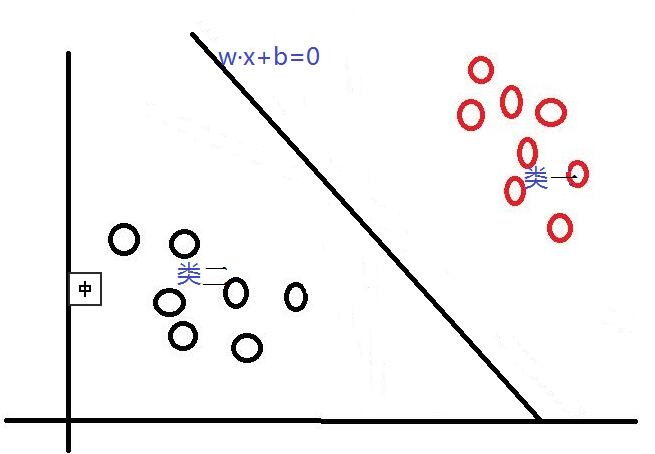其中，w是权重向量，x为训练元组（X=(x1,x2…xn),n为特征个数，xi为每个X在属性i上对应的值），b为偏置，w·x是w和x的点积。当某数据被分类时，就会代入此函数，通过计算f(x)的值来确定所属的类别，当f(x)>0时，此数据被分为类一，当f(x)<0时，此数据被分为类二。通过观察，我们可以发现，如果我们平移或者旋转一下此分类线，同样可以完成数据的分类（如下图），那么，选择哪一个分类线才是最好的呢？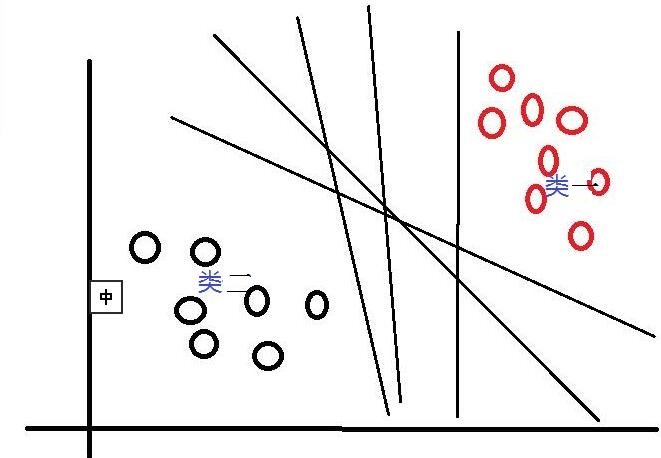对于这个问题，SVM是通过搜索“最大边缘距离分类线”（面）来解决的。那么，什么是边缘距离，为什么要寻找最大的边缘距离呢？如下图所示，如果我们将某一分类线向右平移，在平移到右侧最大限度，又能确保此时的这个被平移的线仍然能将数据分为两类时，也就是如下图（线一）所示的：右侧与类一中某个或某些数据（实心点）相交的位置。此时正好，在线右侧和线上的数据是类一，在线左侧的数据是类二；同理，如果我们将这个分类线向左移动，也是移动到左侧最大限度（如下图线二），此时这条线刚好也与类二中的某个或某些（实心点）数据相交，线上和线左侧的数据是类二，线右侧的数据是类一。对于这两条“极限边界线”，我们可以称之为支持线，或者对于面来说，就是支持面，而确定这些支持面或者支持线的那些数据点，我们称之为支持向量。两个支持线或支持面之间的这个距离，就是我们所说的边缘距离。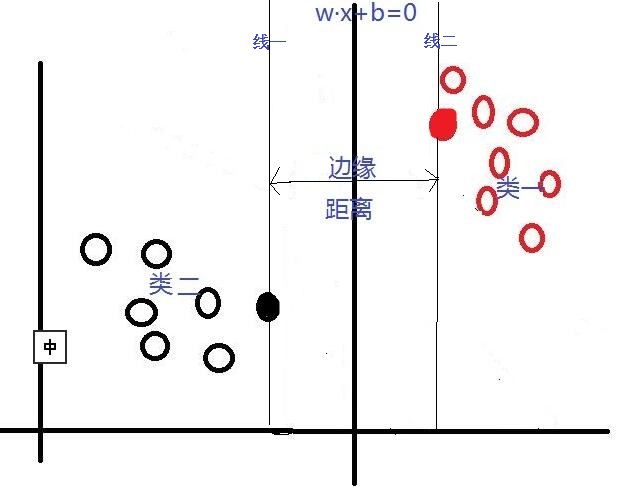在这里我们可以发现，不同的分类线（面）会对应不同的支持线(面），支持线（面）之间的边缘距离也是不同的，并且，我们认为：边缘距离越大的分类线，对分类精度更有保证，所以，我们要找的”最好的“分类线（面），就是拥有最大边缘距离的那个分类线（面）。也就是说：对于线性可分的情况，SVM会选择最大化两类之间边缘距离的那个分类线（面）来完成二分类问题。并且此分类线（面）平行于两个支持线（面），平分边缘距离。下图是一个与上图相比，拥有更大边缘距离的分类线（面）。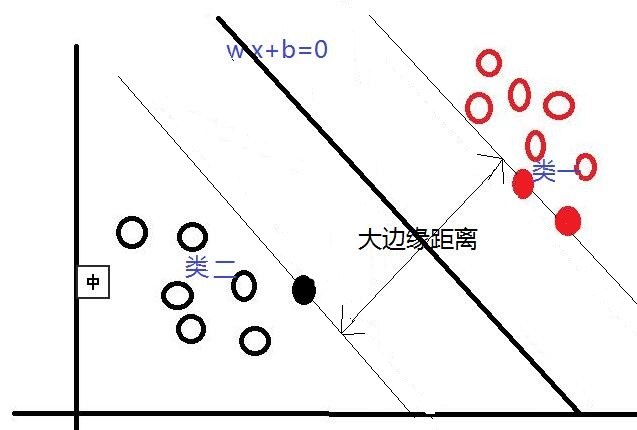2、线性不可分
对于线性可分的情况，我们上面说到，可以通过一个线性函数f(x)=w·x +b来构造一个分类器，寻找一个有着最大边缘距离的分类线（面）来完成对数据的分类。但是，我们还会遇到另一个问题，就是，如果数据是线性不可分的情况，用一个二维直线，三维平面或者多维超平面不能完成二分类，又该如何呢？对于线性不可分问题，SVM采取的方法是将这些线性不可分的原数据向高维空间转化，使其变得线性可分。就像下图所示，对于一些数据，他们是线性不可分的，那么，通过将他们向高维转化，也许就像图中所示，将二维数据转化到三维，就可以通过一个分类面将这些数据分为两类。所以说，SVM通过将线性不可分的数据映射到高维，使其能够线性可分，再应用线性可分情况的方法完成分类。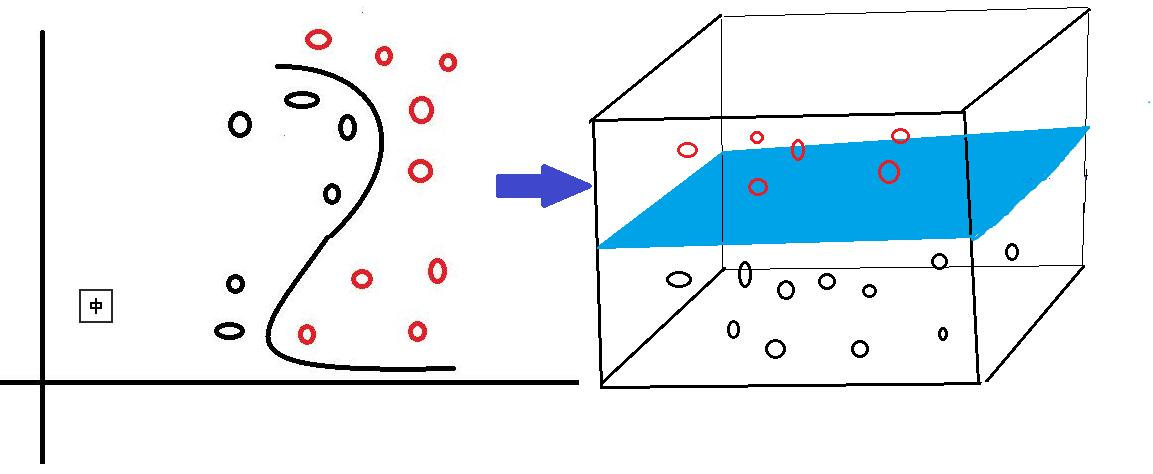而在这个高维转化过程中，SVM实际上并没有真正的进行高维映射，而是通过一种技巧来找出这个最大边缘分类面，即将一个叫做核函数的函数，应用于原输入数据上。这个技巧首先允许我们不需要知道映射函数是什么，只将选定的核函数应用到原输入数据上就行；其次，所有的计算都在原来的低维输入数据空间进行，避免了高维运算。对于这个核函数，可选项有好几种，包括多项式核函数，高斯径向基函数核函数，S型核函数等等。
下面写了两个matlab程序，来简单的看了一下matlab自带的SVM分类器的分类效果。在这里主要应用两个函数来完成而分类问题：
（1)svmtrain函数，其是一个训练分类模型的函数：SVMStruct = svmtrain(Training,Group,Name,Value），其输入参数为（训练数据，训练数据相应组属性，可选参数名，可选参数的值），输出为一个结构体。
可选参数有很多，包括boxconstraint，kernel_function，kernelcachelimit，kktviolationlevel，method，kktviolationlevel，mlp_params，options，polyorder，rbf_sigma，showplot，tolkkt，这里我介绍一下我下面例子中要用到的两个可选输入参数：
2、showplot(绘图）：是一个布尔值，用来指示是否绘制分类数据（这里是训练数据）和分类线。但是这个绘图功能只支持绘制有两个特征值的数据，也就是二维的点数据。（默认为false)，在svmtrain函数中，如果将showplot设置为true，程序会自动在figure中用不同的颜色绘制出训练数据中两个类的点以及通过训练数据获得的分类线，并标注出哪些点是支持向量。（如下图是一组训练数据在通过svmtrain函数训练后，应用showplot绘制出的图形，其中，红色的+代表训练集类1中的数据，绿色的星号代表训练集类2中的数据。支持向量被O圈起。）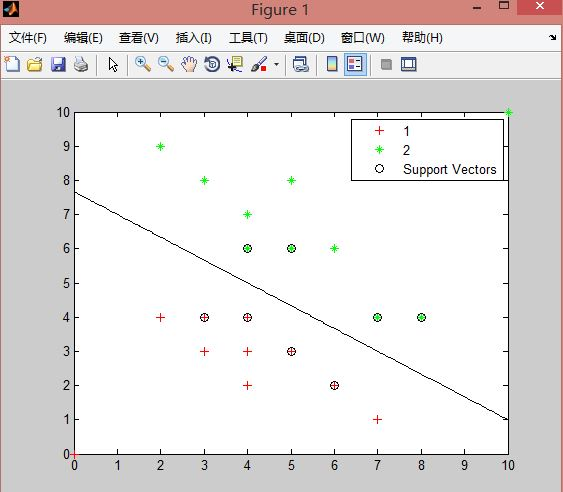3、boxconstraint
svmtrain函数输出的结构体中包含训练出的分类器的信息，包括支持向量机，偏置b的值等等。
（2）svmclassify函数，其实一个应用训练的分类模型和测试数据进行分类测试的函数：Group = svmclassify(SVMStruct,Sample,’Showplot’,true)，其最多只有这四个输入参数，包括（训练出的分类模型结构体，测试数据，绘图显示，’true’）。在svmclassify函数中用Showplot绘图，会绘制出svmclassify函数中输入的测试数据点，如下图所示，粉色的+为被分到类1中的测试数据，蓝色星号是被分到类2中的测试数据）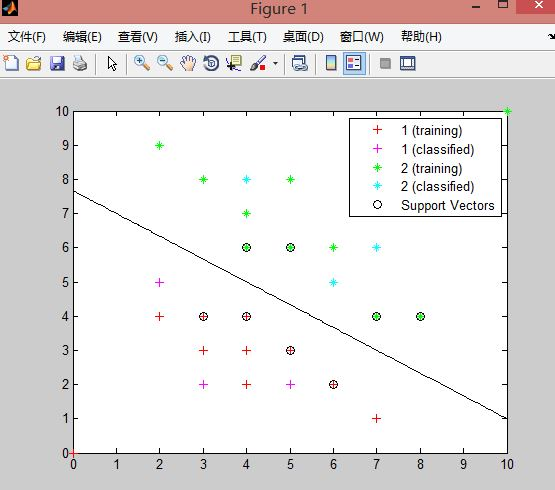对于svmclassify函数的输出Group，其是一个n*1的数组，里面的n为测试数据个数，数组中每个元素记录的是对应顺序下的测试数据被分类后的类属性。
测试1代码

    function [ classification ] = SVM_L( train,test )
%  进行SVM线性可分的二分类处理
%  1、首先需要一组训练数据train，并且已知训练数据的类别属性，在这里，属性只有两类，并用1，2来表示。
%  2、通过svmtrain（只能处理2分类问题）函数，来进行分类器的训练
%  3、通过svmclassify函数，根据训练后获得的模型svm_struct，来对测试数据test进行分类
train=[0 0;2 4;3 3;3 4;4 2;4 4;4 3;5 3;6 2;7 1;2 9;3 8;4 6;4 7;5 6;5 8;6 6;7 4;8 4;10 10];                                              %训练数据点
group=[1,1,1,1,1,1,1,1,1,1,2,2,2,2,2,2,2,2,2,2]'; %训练数据已知分类情况
%与train顺序对应
test=[3 2;4 8;6 5;7 6;2 5;5 2];                                                    %测试数据

%训练分类模型
svmModel = svmtrain(train,group,'kernel_function','linear','showplot',true);

%分类测试
classification=svmclassify(svmModel,test,'Showplot',true);
end
• 1
• 2
• 3
• 4
• 5
• 6
• 7
• 8
• 9
• 10
• 11
• 12
• 13
• 14
• 15
• 16

线性可分实验结果如下图，输出的测试数据分类情况为（1 2 2 2 1 1），全部正确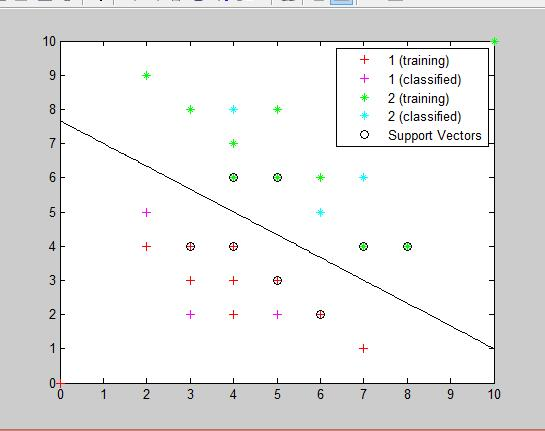测试2代码

function [ classfication ] = SVM_NL( train,tast )
%SVM对线性不可分的数据进行处理
%训练数据
train=[5 5;6 4;5 6;5 4;4 5;8 5;8 8;4 5;5 7;7 8;1 2;1 4;4 2;5 1.5;7 3;10 4;4 9;2 8;8 9;8 10];
%训练数据分类情况
group=[1,1,1,1,1,1,1,1,1,1,2,2,2,2,2,2,2,2,2,2];
%测试数据
test=[6 6;5.5 5.5;7 6;12 14;7 11;2 2;9 9;8 2;2 6;5 10;4 7;7 4];

%训练分类模型
svmModel = svmtrain(train,group,'kernel_function','rbf','showplot',true);
%分类
classification=svmclassify(svmModel,test,'Showplot',true);
end
• 1
• 2
• 3
• 4
• 5
• 6
• 7
• 8
• 9
• 10
• 11
• 12
• 13
• 14
• 15

线性不可分（使用rbf核函数）的实验结果如下图，输出的测试数据分类情况为（1 1 1 2 2 2 2 2 2 1 1），全部正确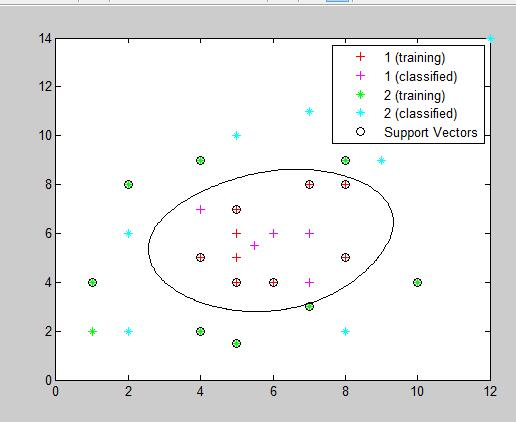因为实验数据是二维的（有两个特征值），可以通过Showplot显示出数据点的分类情况以及最大边界分类线，，且实验数据间干扰较小，分类效果很好。下面的实验将选用matlab提供的一组分类数据来测试一下特征数量对实验精度的影响

测试3代码

function [ classfication ] = SVM2_2( train,test )
%使用matlab自带的关于花的数据进行二分类实验（150*4），其中，每一行代表一朵花，
%共有150行（朵)，每一朵包含4个属性值（特征），即4列。且每1-50，51-100，101-150行的数据为同一类，分别为setosa青风藤类，versicolor云芝类，virginica锦葵类
%实验中为了使用svmtrain（只处理二分类问题）因此，将数据分为两类，51-100为一类，1-50和101-150共为一类
%实验先选用2个特征值，再选用全部四个特征值来进行训练模型，最后比较特征数不同的情况下分类精度的情况。

%和species（150*1 花的类属性数据）
meas=meas(:,1:2);                   %选取出数据前100行，前2列
train=[(meas(51:90,:));(meas(101:140,:))]; %选取数据中每类对应的前40个作为训练数据
test=[(meas(91:100,:));(meas(141:150,:))];%选取数据中每类对应的后10个作为测试数据
group=[(species(51:90));(species(101:140))];%选取类别标识前40个数据作为训练数据

%使用训练数据，进行SVM模型训练
svmModel = svmtrain(train,group,'kernel_function','rbf','showplot',true);
%使用测试数据，测试分类效果
classfication = svmclassify(svmModel,test,'showplot',true);

%正确的分类情况为groupTest，实验测试获得的分类情况为classfication
groupTest=[(species(91:100));(species(141:150))];
%计算分类精度
count=0;
for i=(1:20)
if strcmp(classfication(i),groupTest(i))
count=count+1;
end
end
fprintf('分类精度为：%f\n' ,count/20);

end
• 1
• 2
• 3
• 4
• 5
• 6
• 7
• 8
• 9
• 10
• 11
• 12
• 13
• 14
• 15
• 16
• 17
• 18
• 19
• 20
• 21
• 22
• 23
• 24
• 25
• 26
• 27
• 28
• 29
• 30

选取数据中前两个特征值，进行实验获得的实验精度为0.80，如下图：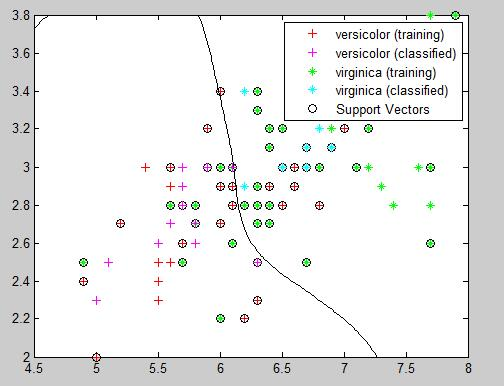将上面实验3中的代码”meas=meas(:,1:2); “改为“meas=meas(:,1:4);” 也就是选取了全部四个特征值，此时的分类结果不能再用showplot打印出，但是，获得的分类精度为1.0 ，这说明，选择适当的特征数量，对分类模型的准确度是有很大影响的。

展开全文• matlab 使用svm进行分类含实例代码（适用于二分类和多分类

# 1. 简单二分类

clear,clc

%% 二分类
%训练数据20×2,20行代表20个训练样本点，第一列代表横坐标，第二列纵坐标
Train_Data =[-3 0;4 0;4 -2;3 -3;-3 -2;1 -4;-3 -4;0 1;-1 0;2 2;3 3;-2 -1;-4.5 -4;2 -1;5 -4;-2 2;-2 -3;0 2;1 -2;2 0];
%Group 20 x 1,20行代表训练数据对应点属于哪一类(1类,-1类)
Train_labels =[1 -1 -1 -1 1 -1 1 1 1 -1 -1 1 1 -1 -1 1 1 1 -1 -1]';
TestData = [3 -1;3 1;-2 1;-1 -2;2 -3;-3 -3];%测试数据
classifier  = fitcsvm(Train_Data,Train_labels); %train
test_labels  = predict(classifier ,TestData); % test


这里 test_labels 就是最后的分类结果啦，大家可以按照这个格式对自己的数据进行修改

# 2. 多分类（不调用工具箱）

因为

%% 多分类
TrainingSet=[ 1 10;2 20;3 30;4 40;5 50;6 66;3 30;4.1 42];%训练数据
TestSet=[3 34; 1 14; 2.2 25; 6.2 63];%测试数据
GroupTrain=[1;1;2;2;3;3;2;2];%训练标签
results =my_MultiSvm(TrainingSet, GroupTrain, TestSet);
disp('multi class problem');
disp(results);


results为最终的分类结果，上述中有用到 my_MultiSvm.m() 函数，以下是my_MultiSvm.m函数的全部内容

function [y_predict,models] = my_MultiSvm(X_train, y_train, X_test)
% multi svm
% one vs all 模型
% Input:
% X_train: n*m矩阵 n为训练集样本数 m为特征数
% y_train: n*1向量 为训练集label，支持任意多种类
% X_test: n*m矩阵 n为测试集样本数 m为特征数
% Output:
% y_predict: n*1向量 测试集的预测结果
%
%

y_labels = unique(y_train);
n_class = size(y_labels, 1);
models = cell(n_class, 1);
% 训练n个模型
for i = 1:n_class
class_i_place = find(y_train == y_labels(i));
svm_train_x = X_train(class_i_place,:);
sample_num = numel(class_i_place);
class_others = find(y_train ~= y_labels(i));
randp = randperm(numel(class_others));
svm_train_minus = randp(1:sample_num)';
svm_train_x = [svm_train_x; X_train(svm_train_minus,:)];
svm_train_y = [ones(sample_num, 1); -1*ones(sample_num, 1)];
disp(['生成模型：', num2str(i)])
models{i} = fitcsvm(svm_train_x, svm_train_y);
end
test_num = size(X_test, 1);
y_predict = zeros(test_num, 1);
% 对每条数据，n个模型分别进行预测，选择label为1且概率最大的一个作为预测类别
for i = 1:test_num
if mod(i, 100) == 0
disp(['预测个数：', num2str(i)])
end
bagging = zeros(n_class, 1);
for j = 1:n_class
model = models{j};
[label, rat] = predict(model, X_test(i,:));
bagging(j) = bagging(j) + rat(2);
end
[maxn, maxp] = max(bagging);
y_predict(i) = y_labels(maxp);
end
end


# 3.多分类(调用libsvm工具箱)

以下代码是调用matlab工具箱libsvm的一种方法

TrainingSet=[ 1 10;2 20;3 30;4 40;5 50;6 66;3 30;4.1 42];%训练数据
TestSet=[3 34; 1 14; 2.2 25; 6.2 63];%测试数据
GroupTrain=[1;1;2;2;3;3;2;2];%训练标签
GroupTest=[1;2;1;3];%测试标签

%svm分类
model = svmtrain(GroupTrain,TrainingSet);
% SVM网络预测
[predict_label] = svmpredict(GroupTest,TestSet,model);


之所以放到最后，是因为需要在matlab安装libsvm的工具箱，具体方法可参看此链接在Matlab中安装LibSVM工具箱

展开全文支持向量机 svm
• 采用MATLAB实现支持向量机（SVM）解决二分类问题，分别采用二次规划凸优化求解、半不无穷规划（线性核与非线性核）求解。 带IRIS数据、实验报告与SVM分类原理数学推导文档，可直接运行，不使用MATLABSVM工具箱...
• 线性支持向量机训练文件 MATLAB代码 可运行
• 文章目录SVM分类对带标签的高光谱数据建立分类模型代码1.训练集、测试集2、数据归一化3、参数选择4、模型训练5、模型测试 SVM分类 采用libSVM包进行多分类 对带标签的高光谱数据建立分类模型 数据描述：三个...
• svm分类器,svm实现多分类,matlab源码
• svm分类器，包括多分类和GA算法和PSO算法优化的SVM
• matlab代码SVM分类器 有关如何在MATLAB中编写SVM分类器的示例代码。 如何运行： 要运行该代码，请创建两个目录来存储两组分类的图像数据。 这些图像目录将用于训练SVM分类器。 然后，将main_script中的两个变量...
• svm分类器，集成了svm多分类解决方案，支持matlab、C、python等
• 简要的matlab基于svm分类的小程序，有三个例子可以作为参考
• 一对多情况下新实例的分类是通过赢家通吃的策略完成的，其中具有最高输出函数的分类器分配类别（重要的是输出函数被校准以产生可比较的分数） . 对于一对一方法，分类是通过最大赢投票策略完成的，其中每个分类器将...
• . function Bayes2 %为了提高实验样本测试的精度故采用多次模拟求平均... %判别矩阵的初始化 for k=1:N %控制程序模拟次数 N %生成二维正态分布的样本 2 X N 维的矩阵 X1=mvnrnd([1 2],[4 0;0 6],300; %2 X N X2=mvnrnd
• 资源名：使用MATLAB语言_利用HOG特征和svm分类器实现行人的检测_matlab 资源类型：matlab项目全套源码 源码说明： 全部项目源码都是经过测试校正后百分百成功运行的，如果您下载后不能运行可联系我进行指导或者...
• ## Matlab-SVM分类器

万次阅读 多人点赞 2016-08-29 11:47:51
支持向量机（Support Vector Machine，SVM)，可以完成对数据的分类，包括线性可分情况和线性不可分情况。... 并用线性函数f(x)=w·x +b来构造这个分类器(如下图是一个二维分界线） 其中，w是权重向量，x为训练元
• SVM分类器的相关算法和matlab源码,部分内容如下，1.命令函数部分： clear;%清屏 clc; X =load('data.txt'); n = length(X);%总样本数量 y = X(:,4);%类别标志 X = X(:,1:3); TOL = 0.0001;%精度要求 C = 1;%参数，...
• 我已经用疲劳和非疲劳样本训练了 SVM 分类器。 怎么跑？？ 1. 解压并把'Sleep'文件夹放在Matlab的路径下。 2. 打开网络摄像头，进入命令窗口并输入“imaqtool”以查找支持的适配器。 3. 打开 main.m 并转到第 1 行...
• [日本人]当由支持向量机 (SVM) 分类时，在 3D 上可视化分离界面。如果有 4 个或更多变量，则无法在 xyz 平面上进行可视化。例如，它是有效的，因为您可以在更改内核类型时直观地检查边界表面如何变化。
• 分类器设计之线性分类器和线性SVM(Matlab代码 具休请参考本人博客： http://blog.csdn.net/ranchlai/article/details/10303031
• 本代码参考 MATLAB 官方例程，实现 SVM分类器（one-versus-rest）。对于未知标签的测试集，初始化中测试样本的标签，以及结果分析中的验证部分可忽略。 OVR SVM 一对多（one-versus-rest）模型的基本策略：训练时......

# svm分类器matlab程序matlab 订阅